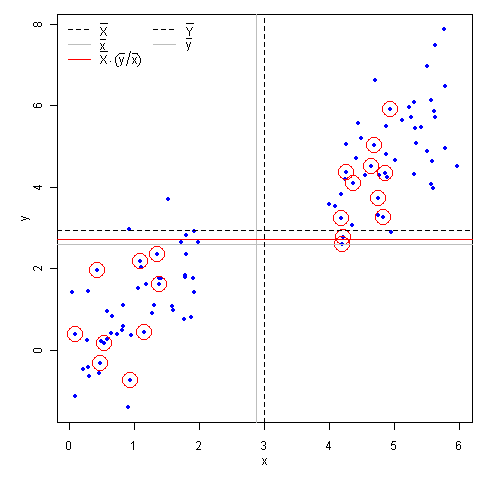# A Demo for the Ratio Estimation in Sampling Survey (Animation)

### Yihui Xie 2010-03-24

Amber Watkins gave me a suggestion on the animation for the ratio estimation, and I think this is a good topic for my animation package. I’ve finished writing the initial version of the function sample.ratio() for this package, which will appear in the version 1.1-2 a couple of days later.

As we know, the benefit of ratio estimation is that sampling skewness may be adjusted for, because the estimation of $$\bar{Y}$$ will make use of the information in the relationship of X and Y: $$\bar{X} \cdot (\bar{y}/\bar{x})$$. Here is a demo (we can see the ratio estimate, denoted by the red line, generally performs better than $$\bar{y}$$):